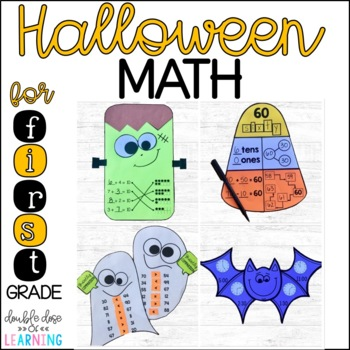# Halloween Math Craftivities for First Grade { Equations, Time, Comparison}K - 1st, Homeschool
Subjects
Standards
Resource Type
Formats Included
• PDF
Pages
34 pages
\$5.50
List Price:
\$6.50
You Save:
\$1.00
\$5.50
List Price:
\$6.50
You Save:
\$1.00

#### Also included in

1. Enhance your students ability to demonstrate their knowledge of first grade math topics by incorporating interactive extensions to your lessons with craftivities or math art. The concepts you will find within this resource are aligned to the common core standards for first grade and some within kind
\$26.00
\$39.00
Save \$13.00

### Description

Enhance and reinforce first grade students ability to demonstrate math skills during October by incorporating interactive Halloween extensions to your lessons with craftivities or math art. Skills include making numbers with place value, number words and number bonds, time to the hour and half hour, comparing numbers 1-100 and missing addends. The concepts you will find within this resource are aligned to the common core standards for first grade and some within kindergarten as well. You will find all you need for 4 different easy-to-prep craftivities which will make the perfect Halloween hallway or bulletin board display!

*Candy Corn Making Numbers (Number words, number bonds, place value and equations)

*Batty for Time (time to the hour and half hour)

*Ghostly Comparison (comparing numbers 1-100)

Halloween Crafts are also available for 2nd Grade Math and ELA for easy differentiation!

Interested in more Math and ELA Craftivities? Available for EVERY major Holiday and Season!

Craftivity BUNDLES (savings of over 20% off!):

Craftivity Individual Units (4 crafts included in each set)

Join our E-Mail list here for teaching tips and strategies, giveaways, freebies and more!

Total Pages
34 pages
N/A
Teaching Duration
N/A
Report this Resource to TpT
Reported resources will be reviewed by our team. Report this resource to let us know if this resource violates TpT’s content guidelines.

### Standards

to see state-specific standards (only available in the US).
Determine the unknown whole number in an addition or subtraction equation relating three whole numbers. For example, determine the unknown number that makes the equation true in each of the equations 8 + ? = 11, 5 = ▯ - 3, 6 + 6 = ▯.
Understand the meaning of the equal sign, and determine if equations involving addition and subtraction are true or false. For example, which of the following equations are true and which are false? 6 = 6, 7 = 8 - 1, 5 + 2 = 2 + 5, 4 + 1 = 5 + 2.
Add and subtract within 20, demonstrating fluency for addition and subtraction within 10. Use strategies such as counting on; making ten (e.g., 8 + 6 = 8 + 2 + 4 = 10 + 4 = 14); decomposing a number leading to a ten (e.g., 13 - 4 = 13 - 3 - 1 = 10 - 1 = 9); using the relationship between addition and subtraction (e.g., knowing that 8 + 4 = 12, one knows 12 - 8 = 4); and creating equivalent but easier or known sums (e.g., adding 6 + 7 by creating the known equivalent 6 + 6 + 1 = 12 + 1 = 13).
Relate counting to addition and subtraction (e.g., by counting on 2 to add 2).
Apply properties of operations as strategies to add and subtract. If 8 + 3 = 11 is known, then 3 + 8 = 11 is also known. (Commutative property of addition.) To add 2 + 6 + 4, the second two numbers can be added to make a ten, so 2 + 6 + 4 = 2 + 10 = 12. (Associative property of addition.)